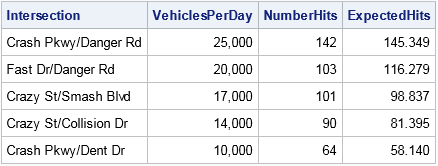How do you sample with replacement in SAS when the probability of choosing each observation varies? I was asked this question recently. The programmer thought he could use PROC SURVEYSELECT to generate the samples, but he wasn't sure which sampling technique he should use to sample with unequal probability. This article describes how to sample with replacement and unequal probability in SAS.

### Sample with replacement and unequal probability with PROC SURVEYSELECT

The programmer's confusion is completely understandable. The SURVEYSELECT documentation is written for survey statisticians, who use specialized language to describe sampling methods. To a survey statistician, sampling with unequal probability is known as sampling with probability proportional to size (PPS), which is often abbreviated as PPS sampling. The SURVEYSELECT procedure has many methods for PPS sampling. For PPS sampling with replacement, specify the METHOD=PPS_WR option.

Sampling in proportion to size has many applications. For example, if you want to survey people at your company, you could randomly select a certain number of people in each building, where the probability of selection is proportional to the number of people who work in each building. Or you could use PPS sampling to obtain a representative sample across departments.

The following example demonstrates sampling with replacement and with unequal probability. Suppose a small town has five busy intersections. The town planners believe that the probability of an accident at an intersection is proportional to the traffic volume. They want to simulate the locations of 500 accidents by using PPS sampling with replacement, where the relative traffic volumes determine the probability of an accident's location.

The following data shows the annual average daily traffic data for each intersection. The call to the SURVEYSELECT procedure uses METHOD=PPS_WR and N=500 to simulate 500 accidents for these intersections. The the SIZE statement specifies the relative traffic, which determines the probability of an accident in each intersection.

```data Traffic; label VehiclesPerDay = "Average Annualized Daily Traffic"; input Intersection \$21. VehiclesPerDay; format VehiclesPerDay comma.; datalines; Crash Pkwy/Danger Rd 25000 Fast Dr/Danger Rd 20000 Crazy St/Smash Blvd 17000 Crazy St/Collision Dr 14000 Crash Pkwy/Dent Dr 10000 ;   /* sample with replacement, probability proportional to size */ proc surveyselect noprint data=Traffic out=Sample method=PPS_WR seed=123 N=500; /* use OUTHITS option if you want 500 obs */ size VehiclesPerDay; /* specify the probability variable */ run;   proc print data=Sample noobs; var Intersection VehiclesPerDay NumberHits ExpectedHits; run;```As you can see, the counts of crashes in the simulated sample are close to their expected values. Each time you run a simulation you will get slightly different values. You can use the REPS= option in the PROC SURVEYSELECT statement to generate multiple samples.

I have blogged about this technique before, but my previous focus was on simulating data from a multinomial distribution. The METHOD=PPS_WR option generates counts that follow a multinomial distribution with proportion equal to the standardized "size" variable (which is VehiclesPerDay / sum(VehiclesPerDay)).

If you want a data set that contains all 500 draws, rather than the counts, you can add the OUTHITS option to the PROC SURVEYSELECT statement.

### Sample with replacement and unequal probability with PROC IML

For SAS/IML programmers, it might be more convenient to simulate data within PROC IML. The SAS/IML language provides two routines for sampling with replacement and with unequal probability. If you want only the counts (as shown in the previous example) you can use the RANDMULTINOMIAL function and specify the proportion of the traffic that passes through each intersection, as follows:

```proc iml; call randseed(54321); use Traffic; read all var {"Intersection" "VehiclesPerDay"}; close; Proportion = VehiclesPerDay / sum(VehiclesPerDay); Counts = RandMultinomial(1, 500, Proportion); /* 1 sample of 500 events */```

If instead you want the full sample (all 500 values in a random ordering), you can use the SAMPLE function. The third argument to the SAMPLE function enables you to specify whether the sampling is done with or without replacement. The fourth argument enables you to specify the unequal sampling probabilities, as follows:

`Sample = sample(Intersection, 500, "Replace", Proportion);`

In summary, when you want to sample with replacement and with unequal probabilities, use the METHOD=PPS_WR option in PROC SURVEYSELECT or use the SAMPLE function in SAS/IML.

Share